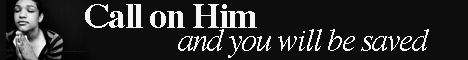Note:  Do not rely on this information. It is very old.

# Mean

Mean, in mathematics, is a sum of a series which comes between two other sums and bears a certain relation to them. The arithmetic mean of two numbers is half their sum, the geometric mean is the square root of their product, and the harmonic mean is twice the product of the two numbers divided by their sum. Between two given numbers it is possible to insert any number of terms such that they form a series in arithmetical, geometrical, or harmonical progression. The terms so inserted are also called means.